# Subtracting Problems

#### Number of problems found: 496

• TyronTyron attended his online class 2 hours a day. He already attend 1 3/4 hours, how many more hours will Tyron attend his class?
• Denissa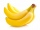Denissa has 18 9/10 kilos of bananas to sell if she was able to sell 15 ⅗. How many kilos of bananas were left?
• 1000 billA hat costs Php 75.25 and a discounted T-shirt costs Php 750.55. How much change will you receive if you pay for both items with a Php 1 000 bill?
• Frame image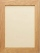Peter wants to put a picture in a frame. The picture is 7 3/5 cm wide. To fit in the picture frame cannot be more than 7 3/10 cm wide. How much should the image be trimmed?
• A rectangle 7A rectangle that has been split into 24 parts, set in 4 rows of 6 parts each. The parts in the top 3 rows have been shaded blue. The parts in the left 5 columns have been shaded purple. The first 5 parts in the top 3 rows are striped to show they are shad
• ShopperEva spent 1/4 in one store and 1/3 in another. What fraction is left?
• Simplify expression with mixedWhen (7¹/3 + 2¾)÷(2 + 2¼ × 1¹/3) is simplified the result is?
• An Argand diagram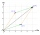Find the sum z1+z2 and difference z1-z2 and z2-z1 of z1=5+2i and z2=2+3i by an Argand diagram.
• Evaluate 17Evaluate 2x+6y when x=- 4/5 and y=1/3. Write your answer as a fraction or mixed number in simplest form.
• A burger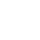The water starts with 41 1/4 cups of water how much water is left after ten 3 5/8 Scoops of water are removed?
• Evaluate fractionsThe difference  of 5 1/2 and 2/3 is added to the product of 5/6 and 1/2
• Fraction expressionWhich expression is equivalent to : minus 9 minus left parenthesis minus 4 start fraction 1 divided by 3 end fraction right parenthesis
• Sum and productThe sum of the real numbers x and y is 12. Their difference is 8. What is the value of xy?
• Kilos of meat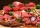Marry bought 6 1/3 kg of meat. She used 2 1/4 kg for Anita 2 1/8 kg for Maros and the rest for Adam. How many kilograms of meat did she used for Adam?
• Ferdinand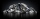Ferdinand went from place A to place B and from there to place C. A is 7.2 km from B and B is 11.7 km from C. Albert went from place A to place D and from there to place C. D is 9.1 km from A and C is 10.8 km from D. Who traveled more and by how much?
• DuringDuring a week, a student's spends 1/3 of her time in bed, 5/24 of her time in lesson and 1/8 of her time doing homeworks. What fraction of her time is left for doing other things?
• A rectangular 4A rectangular garden has a length of 57 m and a width of 42 m. Calculate of how many m2 will decrease the area of a garden, if the ornamental fence with a width of 60 cm will be planted inside its perimeter.
• A farmer 3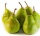A farmer sells 9.3 kg of pears and apples at the farmers market.3/4 of this weight is pears, and the rest is apples. How many kilograms of apples did she sell at the farmers market.
• Evaluate - lowest termsEvaluate: 16/25 - 11/25 (Express answer as a fraction reduced to lowest terms. )
• Sum of 20Sum of the two numbers is 15 1/6. If one of the numbers is 2 2/3, find the other number.

Do you have an exciting math question or word problem that you can't solve? Ask a question or post a math problem, and we can try to solve it.

We will send a solution to your e-mail address. Solved examples are also published here. Please enter the e-mail correctly and check whether you don't have a full mailbox.

Please do not submit problems from current active competitions such as Mathematical Olympiad, correspondence seminars etc...